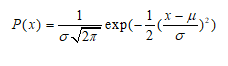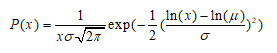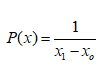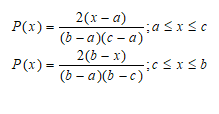# Probabilistic evaluations

In a typical assessment, ConsExpo nano performs what are often called ‘deterministic’ evaluations: the user specifies single values for the different input parameters and ConsExpo evaluates a single number for the exposure.

In addition to this, ConsExpo nano also facilitates probabilistic evaluations. The user may specify probability distributions as parameter input. Whenever distributions have been specified for input, ConsExpo conducts a Monte Carlo simulation. Rather than calculating exposure only once, ConsExpo nano repeatedly samples the input distributions of all distributed parameters. With each sample the inhaled and deposited dose are evaluated, yielding a distribution of calculated outputs. This distribution is then presented as the outcome of the evaluation.

In sampling the distributions of input parameters, ConsExpo nano observes limits of validity on the parameters. If samples of parameter inputs are encountered with one or more parameter values outside the validity domain (e.g. negative body weight, a weight fraction larger than 1), the sample is discarded and a new sample is drawn.

ConsExpo nano supports the following distributions:

Normal

Requires the mean µ and standard deviation σ as input. The distribution is defined by the probability density function:Log Normal

Requires the median µ andgeometric standard deviation (GSD) as input. The probability density is defined as:and

σ=log⁡(GSD)

Uniform

Input is required on a lower bound xo and an upper bound x1 of the distribution. The probability density is defined as:Triangular

Requires as input (a) location (minimum), (b) scale (maximum) and (c) shape (maximal probability). The probability density varies between the minimum and maximum values and has maximum probability at the ‘shape’. It is defined as:Beta

The Beta distribution can be used to specify probabilities between 0 and 1. Specification requires input on two positive shape parameters α and β.

For guidance on the use of the Beta-distribution see, for example (Information Technology Laboratory, 2012) .